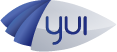#API Docs for: 0.2.0
Show:

# Q.Matrix2D Class

Extends Q.Class
Module: Quintus

A 2D matrix class, optimized for 2D points, where the last row of the matrix will always be 0,0,1

Do not call `new Q.Matrix2D` - use the provided Q.matrix2D factory function for GC happiness

`` var matrix = Q.matrix2d();``

Used internally by Quintus for all transforms / collision detection. Most of the methods modify the matrix they are called upon and are chainable.

## Methods

### `clone`

(
• `matrix`
)
chainable

multiply two matrices (leaving the result in this)

a b = [ [ a11b11 + a12b21 ], [ a11b12 + a12b22 ], [ a11b31 + a12b32 + a13 ] , [ a21b11 + a22b21 ], [ a21b12 + a22b22 ], [ a21b31 + a22*b32 + a23 ] ]

#### Parameters:

• `matrix` Q.Matrix2D
• matrix to multiply by

### `clone`

(
• `matrix`
)
chainable

Clone another matrix into this one

### `extend`

(
• `className`
• `properties`
• `[classMethods]`
)

Create a new Class that inherits from this class

#### Parameters:

• `className` String
• `properties` Object
• hash of properties (init will be the constructor)
• `[classMethods]` Object optional
• optional class methods to add to the class

### `identity`

() chainable

Turn this matrix into the identity

### `isA`

(
• `className`
)

See if a object is a specific class

#### Parameters:

• `className` String
• class to check against

### `release`

()

Release this Matrix to be reused

### `rotate`

(
• `radians`
)
chainable

#### Parameters:

• `radians` Float
• angle to rotate by

### `rotateDeg`

(
• `degrees`
)
chainable

Helper method to rotate by a set number of degrees (calls rotate internally)

#### Parameters:

• `degrees` Float

### `scale`

(
• `sx`
• `[sy]`
)
chainable

Multiply this matrix by a scaling matrix scaling sx and sy

#### Parameters:

• `sx` Float
• scale in x dimension (scaling is uniform unless `sy` is provided)
• `[sy]` Float optional
• scale in the y dimension

### `setContextTransform`

(
• `ctx`
)

Set the complete transform on a Canvas 2D context

#### Parameters:

• `ctx` Context2D
• 2D canvs context

### `transform`

(
• `x`
• `y`
)

Transform x and y coordinates by this matrix Memory Hoggy version, returns a new Array

#### Parameters:

• `x` Float
• `y` Float

### `transformArr`

(
• `inArr`
• `outArr`
)
Object

Transform an array with an x and y elements by this Matrix and put the result in the outArr

#### Parameters:

• `inArr` Array
• input array
• `outArr` Array
• output array

Object:

obj

### `transformPt`

(
• `obj`
)
Object

Transform an object with an x and y property by this Matrix

#### Parameters:

• `obj` Object

Object:

obj

### `transformX`

(
• `x`
• `y`
)
Float

Return just the x coordinate transformed by this Matrix

#### Parameters:

• `x` Float
• `y` Float

Float:

x transformed

### `transformY`

(
• `x`
• `y`
)
Float

Return just the y coordinate transformed by this Matrix

#### Parameters:

• `x` Float
• `y` Float

Float:

y transformed

### `translate`

(
• `tx`
• `ty`
)
chainable

Multiply this matrix by a translation matrix translate by tx and ty

#### Parameters:

• `tx` Float
• `ty` Float